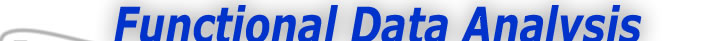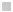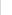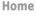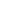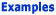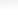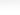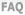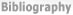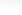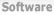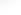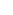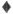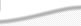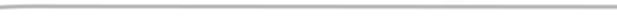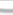BasisFunctions Goods Growth -When do I need monotone curves? -Can I see an example? Weather
 Monotone Smoothing of the Growth Data Previous - 1 - 2 - Next: Can I See an Example? Expertise: Advanced When do I need monotone curves? We often know that the process behind the raw data is always increasing or always decreasing. For example, children and adolescents do not actually lose height, although actual height measurements may fail to increase due to measurement error. Time itself always increases, and any meaningful transformation of time should also increase. Sometimes we need to transform data in a way that ensures that we can reverse the transformation to recover data values in the original scale. This implies that the transformation should be strictly monotone. Monotonicity is a global smoothing principle in the sense that eliminating negative first derivatives can stabilize curves, and especially at the upper and lower boundaries of the independent variable. Why are the usual smoothing curves hard to keep monotone? Linear combinations are by their nature unruly. Even if the basis functions themselves are well behaved, it is hard to prevent certain combinations of coefficients from making the functional data object that they define non-monotone, non-positive or in some way inadmissible. We transform the problem. Instead of using a functional data object to directly fit the data, we use it to define a function that will fit the data and have the desired properties, in this case monotonicity. Suppose W(t) is a conventional functional data object, and that is unconstrained in any way except for W(t0) = 0 where t0 is the lower boundary over which we are smoothing. Then exp[W(t)] is certainly a positive function. And the indefinite integral of a positive function is always increasing. Consequently, we define a monotone smoothing function m(t) to be of the form m(t) = β0 + β1 ∫tt0 exp[W(u)] du In this equation, β0 and β1 are constants that may or may not need to be estimated from the data. β0 is the value of m(t0) and β1 is the slope of m(t) at t0. In addition to these two constants, we need to estimate the coefficients that define the linear combination of basis functions that defines W(t). As a rule, a B-spline basis will be used, and the constraint W(t0) = 0 can easily be achieved by fixing the first coefficient to be zero. The estimation necessarily involves numerical optimization of a fitting criterion, and will therefore involve considerably more computation than the usual data smoothing process. Previous - 1 - 2 - Next: Can I See an Example?